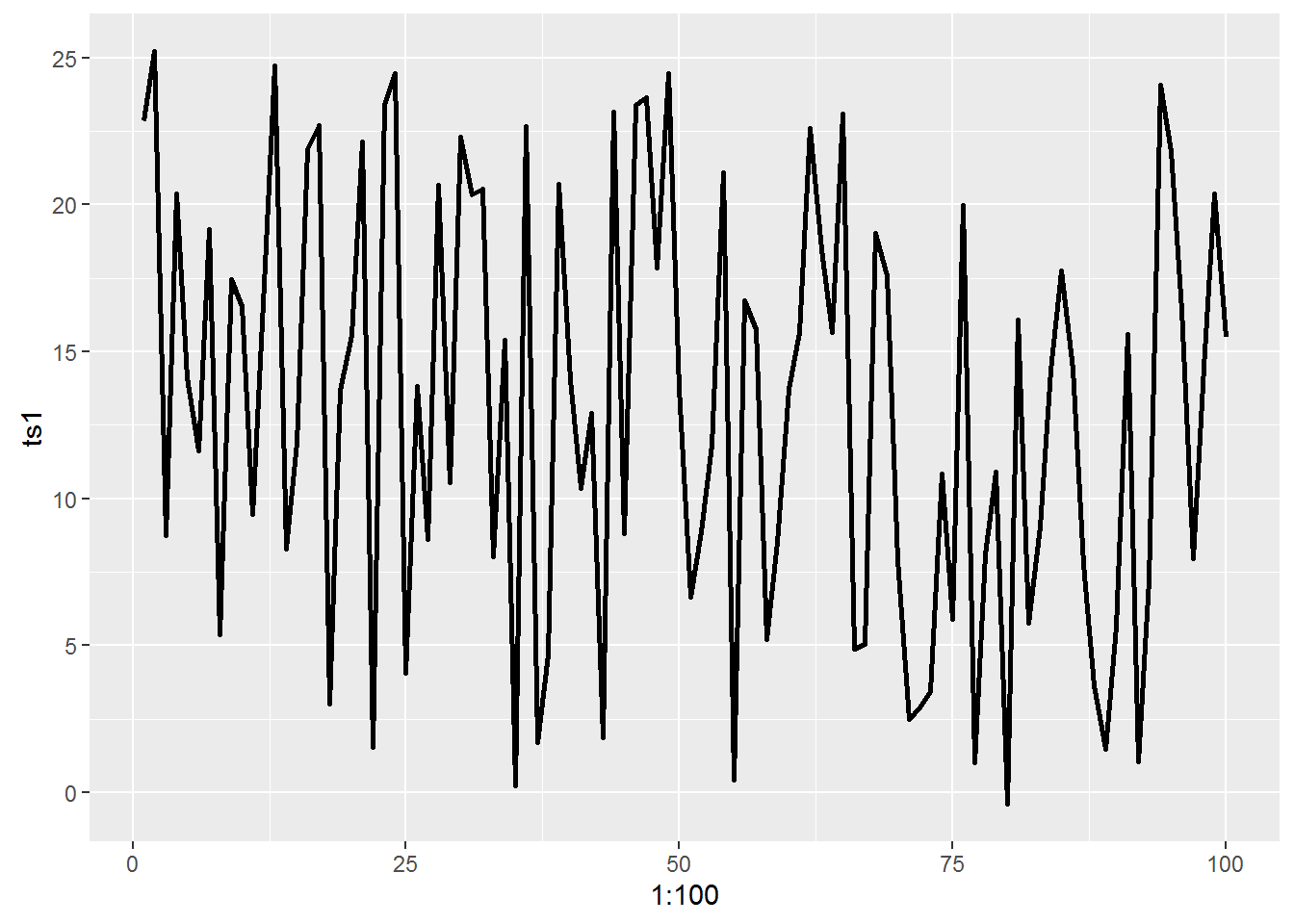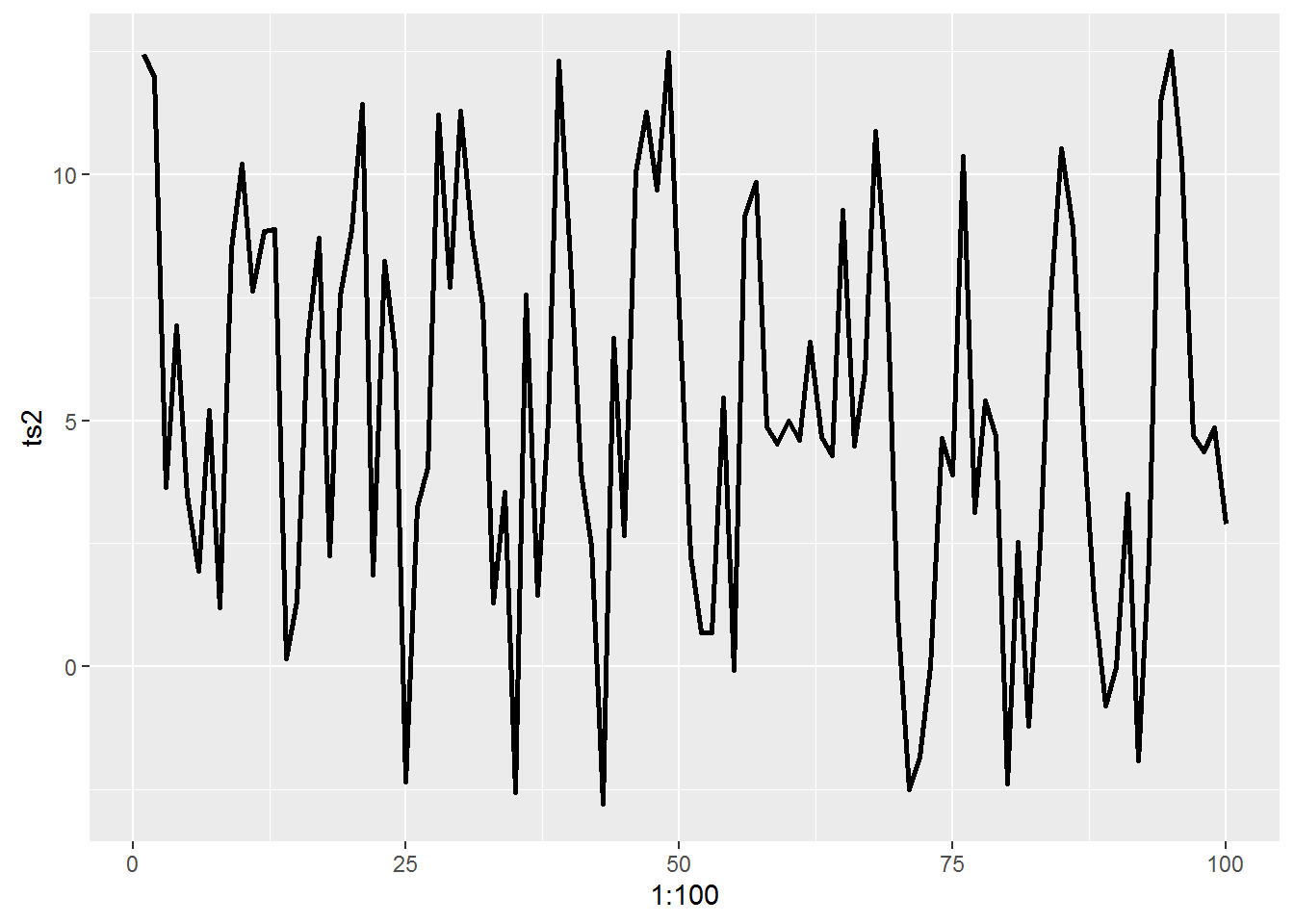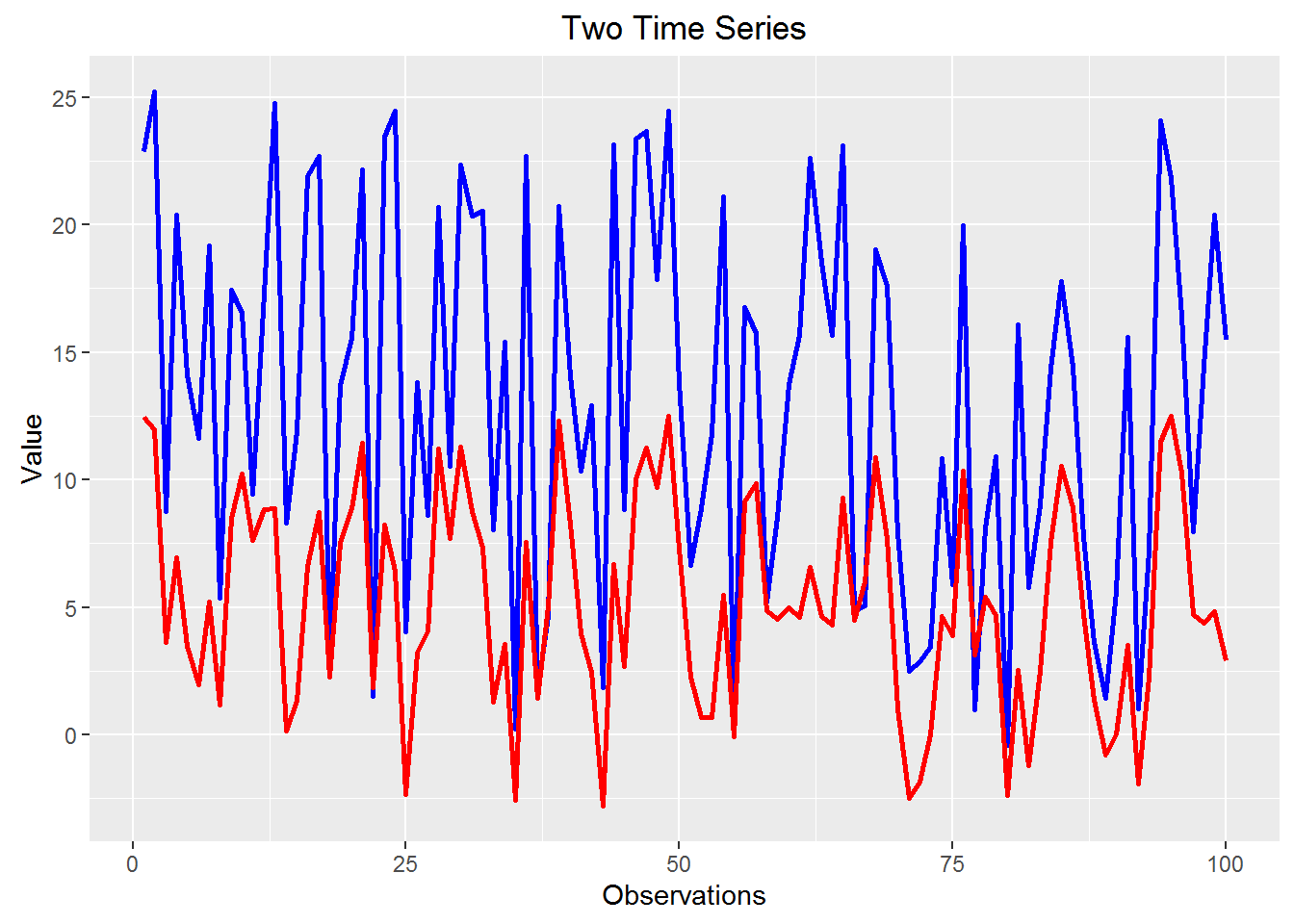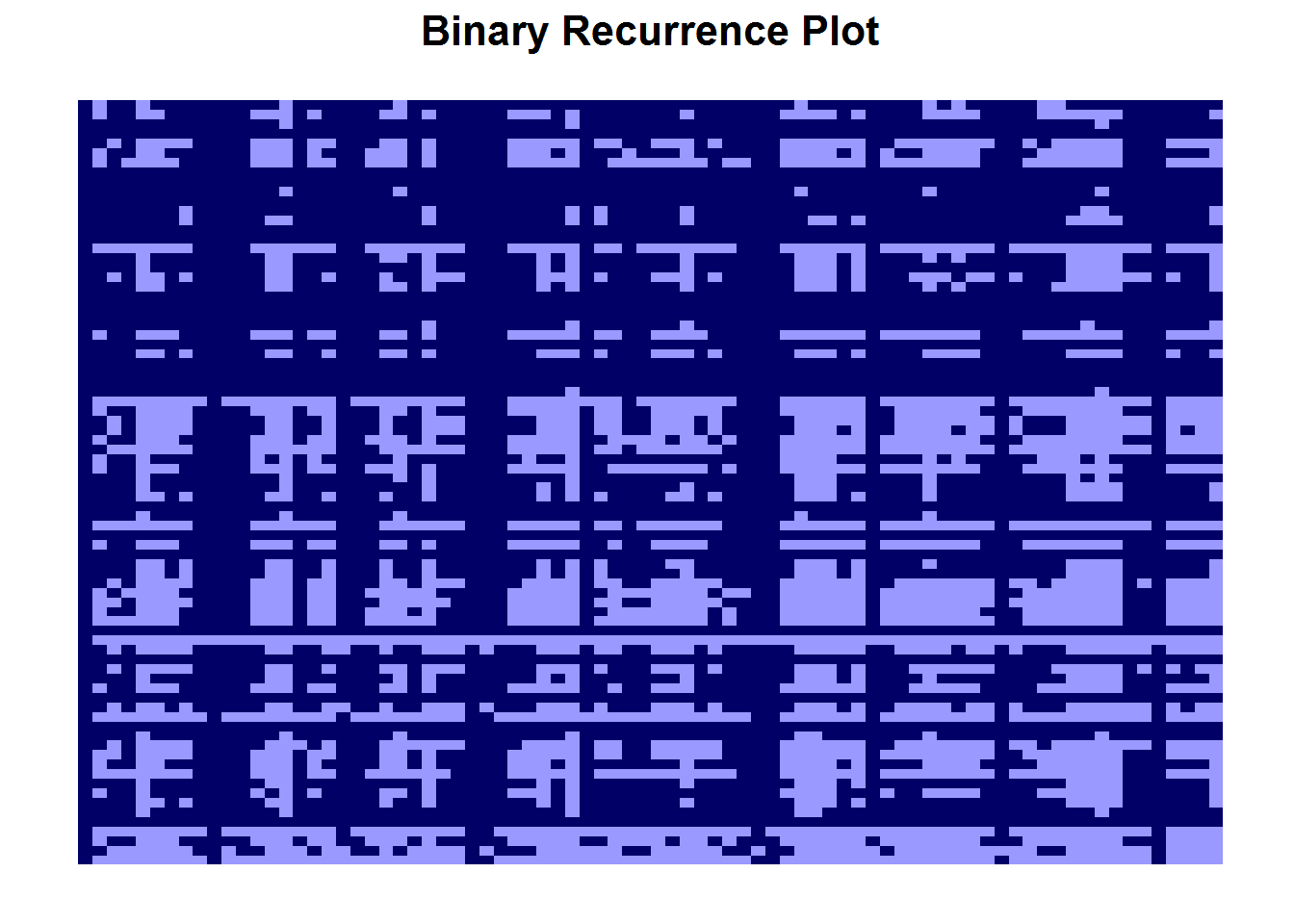### Overview

This tutorial is a general introduction to cross recurrence quantification analysis using the ‘crqa’ package. We will use simulated data for demonstration. However, feel free to substitute with your own data.

### Goals

1. Carry out cross recurrence quantifucation analysis.
2. Understand how to interpret the results.
3. Plot results.

### General Steps

1. Acquire time series and graph to eyeball patterns.
2. Determine hyperparameters.
3. Calculate percent recurrence, percent determinism, and longest line.
4. Create recurrence plot.
5. Carry out any further statistical test using metrics from step 3.

Before we dive in, let’s load all necessary libraries.

``````library(crqa)
library(ggplot2)
library(reshape2)
library(tseriesChaos)
library(gridExtra)``````

#### Step 1: Acquiring and graphing time series

First we will simulate some cyclical data and add in a bit of noise. For CRQA and RQA, data must be in long format. Data may either be categorical or continuous. This tutorial will focus on continuous data.

``````set.seed(42)
t <- seq(0,7*pi,,100)
noise <- runif(100, max = 5)

ts1 <- 2*sin(5*t) + noise*5
ts2 <- 3.3*cos(3*t) + noise*2

df <- data.frame(cbind(ts1,ts2))``````

Let’s graph our first time series

``ggplot(data = df, aes(y = ts1, x = 1:100)) + geom_line(size = 1)``Now, the second.

``ggplot(data = df, aes(y = ts2, x = 1:100)) + geom_line(size = 1)``Similarly, we can overlay the two to see how they behave over time.

``ggplot(data = df) + geom_line(aes(y=ts1, x = 1:100), color = "blue", size = 1) + geom_line(aes(y = ts2, x = 1:100), color = "red", size = 1) + xlab("Observations") + ylab("Value") + ggtitle("Two Time Series")``Now, we want to investigate how these two interact in the same system through cross recurrence quantification (crqa). Alternatively, we can examine the dynamics of the individual time series compared to itself via recurrence quantification analysis (rqa). RQA requires only one time series while crqa requires two simultaneous time series. We will start by exmaining the two recurrence of the two time series together using crqa.

#### Step 2: Determine hyperparameters

As discussed in Brick, Gray, & Staples (in process), crqa requires two time series. In addition, three hyper parameters must be set/determined beforehand: radius, delay, and embedding dimension.

Radius – cutoff boundary that will determine if two points are recurrent or not Delay – how many points to consider when looking for recurrence Embedding Dimension – lag unit

If your data is categorical (and numerically coded), we recommend setting the radius between 0 and 1. This way, each category is identified separate from the others.

Within the ‘crqa’ package, the funtion ‘optimizeParam’ will find the hyperparameters that will lead to the largerst percent recurrence. Hyperparameters can also be fixed. We recommend fixing parameters based on theory when comparing across people or groups.

``````mlpar = list(lgM =  35, radiusspan = 100, radiussample = 100, normalize = 0, rescale = 1, mindiagline = 2,
minvertline = 2, tw = 0, whiteline = FALSE, recpt = FALSE, fnnpercent = 10, typeami = "maxlag")

optpar <- optimizeParam(ts1,ts2,mlpar); optpar``````
``````## \$radius
##  18.02138
##
## \$emddim
##  2
##
## \$delay
##  20``````

#### Step 3: Calculate percent recurrence, percent determinism, and longest line

Now we will calculate percent recurrence, percent determinism, and the length of the longest line using the ‘crpa’ function in the ‘crqa package. We recommend setting ’rescale’ and ‘normalize’ to 0 (do nothing). ‘Rescale’ will apply transformations to the distance matrix and ‘normalize’ will transform the time series. These are customizeable settings, and you have the option to rescale and transform based on your data.

``````ans <- crqa(ts1, ts2, delay = optpar\$delay, embed = optpar\$emddim, radius = optpar\$radius, rescale = 0, normalize = 0, mindiagline = 2, minvertline = 2)

metrics <- c(percentRecurrence = ans, percentDeterminism = ans, longestLine = ans); metrics``````
``````## \$percentRecurrence.RR
##  66.48438
##
## \$percentDeterminism.DET
##  89.23619
##
## \$longestLine.L
##  4.574699``````

If we had only time series, we could easily perform recurrence quantification analysis. We would compare ts1 to ts1. With only one time series, hyperparameters can still be optimized and the ‘crqa’ function works in the same way.

``rqaPars <- optimizeParam(ts1,ts1,mlpar); rqaPars``
``````## \$radius
##  17.37764
##
## \$emddim
##  2
##
## \$delay
##  20``````
``````rqaAns <- crqa(ts1, ts1, delay = rqaPars\$delay, embed = rqaPars\$emddim, radius = rqaPars\$radius, rescale = 0, normalize = 0, mindiagline = 2, minvertline = 2)

rqaMetrics <- c(rqaAns, rqaAns, rqaAns); rqaMetrics``````
``````## \$RR
##  69.8125
##
## \$DET
##  90.82363
##
## \$L
##  4.213915``````

#### Step 4: Drawing the recurrence plot.

The plots produced by crqa are a visually appealing method to see the underlying dynamics change over time. We will make two plots: a binary recurrence plot and a contour plot.

First, this binary plot applies the cutoff radius to determine if values in the distance matrix are recurrent or not.

``````mRP <- melt(as.matrix(ans\$RP), varnames=c("TimeV1", "TimeV2"), value.name="Recurrence")

binary <- ggplot(mRP, aes(x=TimeV1, y=TimeV2, fill=Recurrence)) +
geom_raster() +
theme(axis.line = element_blank(),
panel.grid.major = element_blank(),
panel.grid.minor = element_blank(),
panel.background = element_blank(),
axis.text  = element_blank(),
axis.ticks = element_blank(),
axis.title = element_blank()) +
ggtitle("Binary Recurrence Plot") +
scale_fill_manual(values=c("#9999ff","#000066"),
breaks=c(TRUE, FALSE))+
theme(legend.position="none", plot.title = element_text(size = 16, face = "bold"))
binary``````The contour plot shows a gradient of radii so that the distances are more visible. Darker areas indicate areas that are more likely to be considered recurrent. The binary plot shows recurrence once a cutoff radius has been set. Therefore, dark areas are recurrent given the hyperparameters used in Step 3.

``````xyz1 <- embedd(window(ts1, start = 1, end = 100),
m = optpar\$emddim, d = optpar\$delay) # NB: cannot handle an embedding dimension of 1 even if that was the optimized value

xyz2 <- embedd(window(ts2, start = 1, end = 100),
m = optpar\$emddim, d = optpar\$delay) # NB: cannot handle an embedding dimension of 1 even if that was the optimized value

whole1 <- rbind(xyz1,xyz2)
D <- dist(whole1)
D <- as.matrix(D)/max(D)

D <- D[nrow(xyz1):nrow(D), 1:nrow(xyz1)]

D <- melt(D)

contour <- ggplot(data = D, aes(x = Var1,y = Var2, z=value)) +
geom_raster(aes(fill = value), interpolate=TRUE) +
scale_fill_gradient2(low = "black", mid = "#0066CC",
high = "white", midpoint = .45, space = "Lab",
na.value = "grey50", guide = "colourbar") +
geom_contour(aes(color=..level..), bins=6, alpha=.7) +
scale_color_gradient2(low = "black", mid = "#0066CC",
high = "white", midpoint = .45, space = "Lab",
na.value = "grey50", guide = "colourbar") +
theme(legend.position="none") +
theme(axis.line = element_blank(),
panel.grid.major = element_blank(),
panel.grid.minor = element_blank(),
panel.background = element_blank(),
axis.text  = element_blank(),
axis.ticks = element_blank(),
axis.title = element_blank()) +
ggtitle("Contour Recurrence Plot") +
theme(plot.title = element_text(size = 16, face="bold"))
contour``````